Courses

# Fajan's Rule and Polarisation JEE Notes | EduRev

## JEE : Fajan's Rule and Polarisation JEE Notes | EduRev

The document Fajan's Rule and Polarisation JEE Notes | EduRev is a part of the JEE Course Chemistry Class 11.
All you need of JEE at this link: JEE

Dipole Moment

• If a covalent bond is formed between two dissimilar atoms, eg. A and B, one of the atoms (A or B) must be more electronegative than the other. If A is more electronegative than the shared pair of electrons is drawn near A leaving a positive charge on B and hence making the molecule dipolar (A–B+). The percentage of polar character is given in terms of dipole moment (μ).
• The dipole moment is defined as the product of electric charge q and the distance r between the two atoms of a polar molecule. (μ = e × d) Dipole moment is a vector quantity with direction same as that of the line joining positive and negative centres.
• Thus molecules having dipole moment (μ = 0) are called non polar molecules and molecules with  > 0 are polar. Greater is μ, greater is the polarity.
• For polyatomic molecules with two or more bonds, net dipole moment is the resultant of vector addition of individual moments.
• While expressing dipole moments, generally charge is given in electrostatic units (esu) and distance in angstrom units (1Aº =10–10 m). Thus dipole moment of an electron separated from unit positive charge by a distance 1Aº would be (4.80 × 10–10 esu) × (10-8 cm) = 4.8 × 10–18 esu cm = 4.8 Debye.

Applications of Dipole Moment

1. Dipole moment is helpful in predicting the geometry of the molecule.
2. Dipole moment helps in determining the polarity.
3. Dipole moment can distinguish between symmetrical and non-symmetrical molecules. eg. CO2 has  0 dipole moment as it is symmetrical whereas H2O has a dipole moment of 1.85D.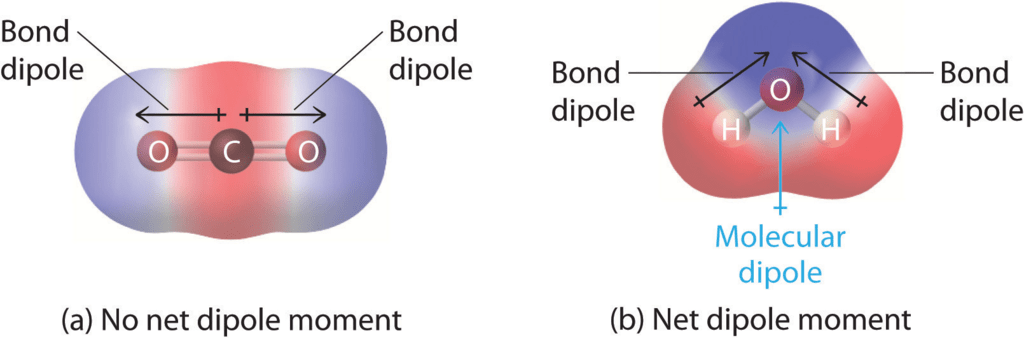1. Cis and trans isomers can be distinguished by dipole moments, usually cis isomers have higher dipole moment and hence higher polarity e.g.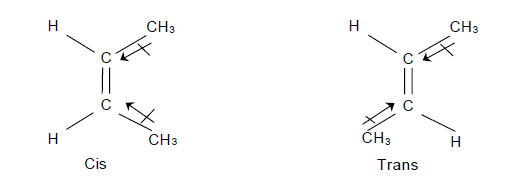1. Dipole moment is greatest for ortho isomer, zero for para isomer and less than that of ortho for meta isomer. o > m > p. e.g.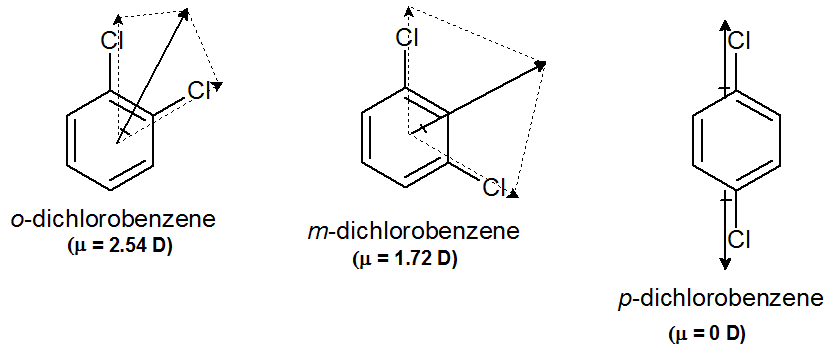1. Ionic character can be determined by using dipole moment
For e.g. experimental dipole moment for HCl is 1.03  and μ = r X e
as r = 1.26 and, e = 4.8 × 10-10 esu,
μ = 6.05 Debye.
Thus the ionic character
Thus we can say % ionic character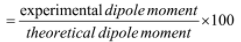1. Hybridisation can be determined by dipole moment for eg.
1. If a molecule AB2 has μ=0, the s orbitals used  by
A (z < 21) must be sp hybridised e.g. BeF2
2. If a molecule AB3 has μ=0, the s orbitals used by
A (z < 21) must be sp2 hybridised e.g. BF3
3. If a molecule AB4 has μ =0, the s orbitals used by
A (z < 21) must be sp3 hybridised e.g. CCl4

Fajan's Rule

Fajans’ rule predicts whether a chemical bond will be covalent or ionic. A few ionic bonds have partial covalent characteristics which were first discussed by Kazimierz Fajans in 1923. At that time with the help of X-ray crystallography, he was able to predict ionic or covalent bonding with the attributes like ionic and atomic radius.

What is Fajans’ rule?

We classify certain compounds as ionic and other compounds as covalent. Now if we were to ask, amongst the alkali chlorides, which is the most ionic? To answer these kinds of questions, we employ Fajans’ rules as a tool.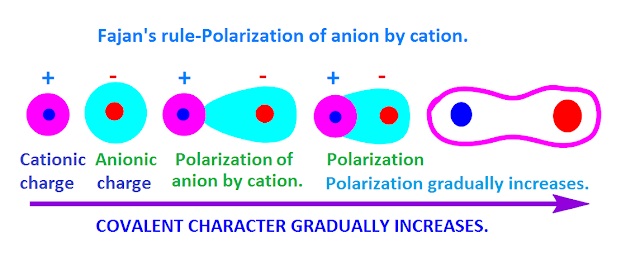Polarising power
It is the extent to which a cation can polarise an anion. It is proportional to charge density. Charge density is the ratio of charge to volume. Polarising power α Charge density. More the charge density, greater is the polarising power for that cation.

Polarisability
It is the extent to which an ion can be polarised. It can also be called as the ease with which an ion can be polarised. Polarisation is the distortion of a spherically symmetric electron cloud to an unsymmetric cloud.
Postulates of Fajans’ Rule
The rule can be stated on the basis of 3 factors, which are:
1. Size of the ion: Smaller the size of cation, the larger the size of the anion, greater is the covalent character of the ionic bond.
2. The charge of Cation: Greater the charge of cation, greater is the covalent character of the ionic bond.
3. Electronic configuration: For cations with same charge and size, the one, with (n-1)dn nso which is found in transition elements have greater covalent character than the cation with ns2 np6 electronic configuration, which is commonly found in alkali or alkaline earth metals.

Explanation of Fazans’ Rule
Rule 1:
The first rule speaks about the polarising power of the cation. If the cation is smaller, then we can say that the volume of the ion is less. If the volume is less, we can conclude that the charge density of the ion would be high.
Since the charge density is high, the polarising power of the ion would be high. This makes the compound to be more covalent.

Rule 2:
The second rule speaks about the polarizability of the anion. Larger the anion, less is the effective nuclear charge that holds the valence electron of the ion in place. Since the last electron is loosely bound in large anions, it can easily be polarised by a cation, thereby making the compound more covalent.

Rule 3:
The third rule is a special case. Let us use an example to explain this point.
Example: If we want to find the more covalent compound among HgCl2 and Calcium Chloride we cannot use size as a factor to conclude. This is because both Hg2+ and Ca2+ are of almost equal size. To explain this, we employ the third rule.
The electronic configuration of Hg2+ is 6s0 5d10. This configuration is called pseudo-octet because d-orbital is fully filled, but the element does not have 8 electrons or an octet.

We know that d orbitals are not good at shielding, so we can say that the anion (Cl–) would be more polarised because the d orbital is poor at shielding making HgCl2 more covalent than CaCl2 because Ca2+ ion has a noble gas configuration.
Now to answer the question that we asked first, amongst the alkali chlorides, which one is the most covalent?
Since the anion is the same, we have to compare the cations. According to Fajans’ rules, smaller the cation, more is the covalency. Therefore, LiCl is the most covalent.

Let us Understand Fajans’ Rule Using a Detailed Illustration:
Consider Aluminum Iodide (AlI3)

This is an ionic bond which was formed by transfer of electrons.

• The iodine being bigger has a lesser effective nuclear charge. Thus, the bonding electrons are attracted lesser towards the Iodine nucleus.
• On the contrary, the aluminium having three positive charges attracts the shared pair of electrons towards itself.
• This leads to insufficient charge separation for it to be ionic and so it results in the development of covalent character in AlI3.

Consider Aluminium Fluoride (AlF3)
This is an ionic bond which was also formed by transfer of electron. But here the fluorine being smaller attracts the shared pair of an electron more towards itself and so there is sufficient charge separation to make it ionic.
Examples on Fajans’ rule

Illustration 1: Which compound should theoretically the most ionic and the most covalent amongst the metal halides?
Sol.

The smallest metal ion and the largest anion should technically be the most covalent
Therefore, LiI is the most covalent.
The largest cation and the smallest anion should be the most ionic. Therefore, CsF should be the most ionic.

Illustration 2: Arrange the following according to the increasing order of covalency:

• NaF, NaCl, NaBr, NaI
• LiF, NaF,KF,RbF,CsF

Sol.
1. Since the cation is the same, compare the anions. Amongst the anions, larger the size more would be the covalency. Therefore the order is: NaF < NaCl < NaBr < NaI
2. Here the anion is the same, so we compare with cations. Smaller the cation more is the covalency. Therefore, the order is: CsF < RbF < KF < NaF < LiF
Fajans’ Rule can be summarized as: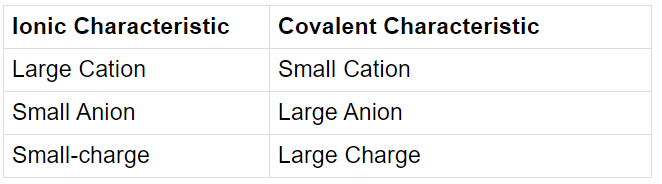Inert Pair Effect

The reluctance of s electron pair of some heavy elements

(p - block elements present at the bottom of group) to take part in bonding is known as inert pair effect. Thus Tl (6s2, 6p1) show +1 electrovalence. Similarly Sn(5s2p2) and Pb (6s2p2) show + 2 oxidation  state commonly. In such elements the lower oxidation state is more stable than higher oxidation state.

Offer running on EduRev: Apply code STAYHOME200 to get INR 200 off on our premium plan EduRev Infinity!

## Chemistry Class 11

136 videos|245 docs|180 tests

,

,

,

,

,

,

,

,

,

,

,

,

,

,

,

,

,

,

,

,

,

;